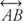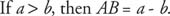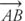## Segments Midpoints and Rays

The concept of lines is straightforward, but much of geometry is concerned with portions of lines. Some of those portions are so special that they have their own names and symbols.

Line segment

A line segment is a connected piece of a line. It has two endpoints and is named by its endpoints. Sometimes, the symbol – written on top of two letters is used to denote the segment. This is line segment CD (Figure 1).Figure 1 Line segment.

It is written CD (Technically, CD refers to the points C and D and all the points between them, and CD without therefers to the distance from C to D.) Note that CD is a piece of.

Postulate 7 (Ruler Postulate): Each point on a line can be paired with exactly one real number called its coordinate. The distance between two points is the positive difference of their coordinates (Figure 2).Figure 2 Distance between two points.Example 1: In Figure 3, find the length of QU.Figure 3 Length of a line segment.Postulate 8 (Segment Addition Postulate): If B lies between A and C on a line, then AB + BC = AC (Figure 4).Figure 4 Addition of lengths of line segments.

Example 2: In Figure 5, A lies between C and T. Find CT if CA = 5 and AT = 8.Figure 5 Addition of lengths of line segments.

Because A lies between C and T, Postulate 8 tells youMidpoint

A midpoint of a line segment is the halfway point, or the point equidistant from the endpoints (Figure 6).Figure 6 Midpoint of a line segment.

R is the midpoint of QS because QR = RS or because QR = ½ QS or RS = ½ QS

Example 3: In Figure 7, find the midpoint of KR .Figure 7
Midpoint of a line segment.The midpoint of KR would be ½(24), or 12 spaces from either K or R. Because the coordinate of K is 5, and it is smaller than the coordinate of R (which is 29), to get the coordinate of the midpoint you could either add 12 to 5 or subtract 12 from 29. In either case, you determine that the coordinate of the midpoint is 17. That means that point O is the midpoint of KR because KO = OR.

Another way to get the coordinate of the midpoint would be to find the average of the endpoint coordinates. To find the average of two numbers, you find their sum and divide by two. (5 + 29) ÷ 2 = 17. The coordinate of the midpoint is 17, so the midpoint is point O.

Theorem 4: A line segment has exactly one midpoint.

Ray

A ray is also a piece of a line, except that it has only one endpoint and continues forever in one direction. It could be thought of as a half‐line with an endpoint. It is named by the letter of its endpoint and any other point on the ray. The symbol → written on top of the two letters is used to denote that ray. This is ray AB (Figure 8).Figure 8 Ray AB.

It is written asThis is ray CD (Figure 9).Figure 9 Ray CD.It is written asorNote that the nonarrow part of the ray symbol is over the endpoint.